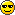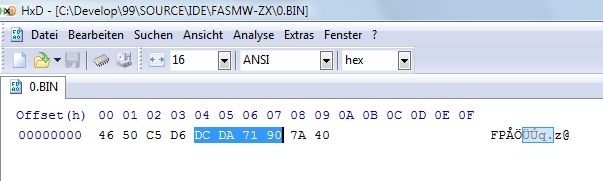flat assembler
Message board for the users of flat assembler.Index > Main > math calculations - converting ascii in floating point
Author
 Threadshutdownall Joined: 02 Apr 2010 Posts: 517 Location: Munich shutdownall Maybe there are some math experts here. I want to change a number based on 10 into floating point format based on 2. This is for the Sinclair BASIC needed. This is not standard format, its a bit better than single precision. The mantissa has 32 bits and the exponent has 8 bits (with bias of 80h). So I now can convert an integer in ascii (1234567890) into 32 bit mantissa. If it is a floating point, it could be formatted in E-format. 12345.67890 = 1234567890E-5. The allowed range is 10**+/-38. What would be the best way to convert a 10-based exponent into a 2-based exponent ? If I convert it fully in binary I need 128 bit calculation but only the first 32 bits (significant bits) are really needed. Any proposals ? And it should run on most x86 computers produced lets say in the last 5 years. SSE5 could handle with 128 bits as I know but this is a feature not widely available. Thanks for any help and comments.27 Jul 2013, 21:12
tthsqe

Joined: 20 May 2009
Posts: 731
tthsqe
You will need to provide more details on the mantissa. Is the leading bit in the mantissa implied? Are negative numbers represented with a two's complement mantissa?28 Jul 2013, 01:11shutdownall Joined: 02 Apr 2010 Posts: 517 Location: Munich shutdownall Well, its quite easy. I have no problem to convert a 10 digit integer into it. For example: 1234567890 I go from first to last digit, multiply contents of edx with 10 and add the digit with an initial value of zero. The exponent is 80h plus length of binary integer, so 5 is 101 which will give an exponent of 83h and after the mantissa is shifted to the left with first beginning binary one at MSB. The integer 5 has mantissa 10100000 :00h:00h:00h=A0000000h. As the binary integer is normalized due to shift to the left as maximum as possible, the first bit is used for positive/negative. It is reset for positive values and set for negative values. The full floating point format is exponent + mantissa in 5 bytes: So 5 is coded as 83 20 00 00 00 in hex bytes. -5 would be 83 A0 00 00 00 0.2 (1/5) would be 7D 20 00 00 00 so in the other direction the exponent is subtracted, 80h -3 = 7Dh instead of 80h +3 = 83h. There is a special coding for zero: 00 00 00 00 00 which is more a special condition than a floating point value. It is more detailed described here (NEW ROM arithmetic): http://www.users.waitrose.com/~thunor/mmcoyzx81/chapter17.html This is my code for converting a positive integer in edx and write floating point via stos: Code:```zx81_numtofp: or edx,edx jne @f xor eax,eax stosb stosd ret @@: bsr eax,edx mov ecx,31 sub ecx,eax add eax,81h stosb or ecx,ecx je zx81_ntf1 shl edx,cl zx81_ntf1: and edx,7fffffffh mov eax,edx bswap eax stosd ret ``` So the question is what is the best/fastest way to convert ascii floating point notations with big exponent base on 10 to this format based on 2 ? Like 5.32999E+29 =5.32999*10**29 ?? As example.28 Jul 2013, 11:13
cod3b453

Joined: 25 Aug 2004
Posts: 618
cod3b453
For 10 base 10 digits, ceil(log2(10^10)) is 34 so using 32bit intermediate for your conversion won't work.

Also the usual format for FP is (-1 * sign bit) * 1.fffff * base^exponent, where the ffff's are fractional bits in the mantissa. So 5 would be +1.01x2^2 or exponent=0x82 and mantissa=0x40000000. As tthsqe said, negative numbers will be a problem; also by having an explicit MSbit for the "1." you lose a bit of precision.

The conversion for 5.32999E+29 would be to take 5.32999 and convert it to float by looking at integer part - i.e. 5 = 101 = 1.01x2^2 therefore 1.3324975x2^2 (this can be done using integer shift on 64bit register).

Now take 10^29 and convert it to base 2 via log rules: log2(10^29)=29*log2(10)=96.33... and so the exponent is 98.33... . Now adjust the mantissa, to get an integer exponent, by multiplying by 2^0.33 i.e. (1.3324975*2^0.33) * 2^98.33/(2^0.33) =1.67496*2^98.28 Jul 2013, 20:35shutdownall Joined: 02 Apr 2010 Posts: 517 Location: Munich shutdownall cod3b453 wrote:For 10 base 10 digits, ceil(log2(10^10)) is 34 so using 32bit intermediate for your conversion won't work. That's why I wrote about 128 bit in the first posting. And please look at the title of my code example: This is my code for converting a positive integer (!) in edx and write floating point via stos cod3b453 wrote: Also the usual format for FP is (-1 * sign bit) * 1.fffff * base^exponent, where the ffff's are fractional bits in the mantissa. So 5 would be +1.01x2^2 or exponent=0x82 and mantissa=0x40000000. As tthsqe said, negative numbers will be a problem; also by having an explicit MSbit for the "1." you lose a bit of precision. The difference is, that Sinclair used an exponent BIAS of 80h while standard IEEE 754 uses a BIAS of 7fh. Anyway my calculation is correct. I did not write something about standard floating point. We are talking here about a BASIC computer from the 80s. cod3b453 wrote: Now take 10^29 and convert it to base 2 via log rules: log2(10^29)=29*log2(10)=96.33... and so the exponent is 98.33... . Now adjust the mantissa, to get an integer exponent, by multiplying by 2^0.33 i.e. (1.3324975*2^0.33) * 2^98.33/(2^0.33) =1.67496*2^98. Nice idea but there is no "log" in the assembler instruction set, isn't it ? I need a program which converts a ascii floating point notation in the Sinclair floating point format (which differs from the IEEE 754 format).29 Jul 2013, 17:03
cod3b453

Joined: 25 Aug 2004
Posts: 618
cod3b453
Your first post made no mention of "positive" and I read your second post as meaning you hadn't considered the negative case. Since I've never seen Sinclair floats, I had no idea that it was an actual convention.

Anyway, just because there's no log instruction doesn't mean you can't calculate it using series expansion: http://mathworld.wolfram.com/SeriesExpansion.html

EDIT: Actually log2(10) is constant so no log calculation is required, just mul.

Last edited by cod3b453 on 29 Jul 2013, 18:26; edited 1 time in total29 Jul 2013, 17:25tthsqe

Joined: 20 May 2009
Posts: 731
tthsqe
This is not complicated, with your description of the format you can do something like:
Code:
```; Input:  esi address of input string
; Output  edi address to write 5 byte result

Parse:    xor  edx,edx  ; integer
xor  ecx,ecx  ; exponent
xor  eax,eax
xor  ebx,ebx
test  al,al
jz  Convert
cmp  al,'.'
je  .dot
sub  al,'0'
js  Error
cmp  al,'9'
ja  Error
imul  edx,10
.dot:    test  ebx,ebx
jnz  Error

Error:   int3

Ten:     dd 10

Convert:   ; st0 = edx / 10^ecx
sub  esp,16
mov  [esp],edx
fild  dword[esp]
fld1
fild  dword[Ten]
test  ecx,ecx
jz  .w3
.w1:      shr  ecx,1
jnc  .w2
fmul  st1,st0
.w2:     test  ecx,ecx
jz  .w3
fmul  st0,st0
jmp  .w1
.w3:     fstp  st0
fdivp  st1,st0

fstp  tword[esp]
mov  eax,dword[esp+4]
movzx  ecx,word[esp+8]
and  ecx,0x7FFFF
jz  .zero

btr  eax,31            ; reset for positive
stosd
mov  eax,ecx
stosb
ret

.zero:    xor  eax,eax
stosd
stosb
ret               ```29 Jul 2013, 18:17shutdownall Joined: 02 Apr 2010 Posts: 517 Location: Munich shutdownall Thanks for your example. Not easy to understand for me right now as I don't used fpu instructions till now. By the way, there should be a way to let FASM convert an ASCII floating point into single precision value ??? Quote: Any numerical expression can also consist of single floating point value (flat assembler does not allow any floating point operations at compilation time) in the scientific notation, they can end with the f letter to be recognized, otherwise they should contain at least one of the . or E characters. So 1.0, 1E0 and 1f define the same floating point value, while simple 1 defines an integer value. I did not used before, maybe anyone could give an example. Because I only need this conversion during compile time of FASM as I use a special version of FASM to generate BASIC code for Sinclair computers. There is no calculation necessary, just convert ascii float to single precision format. I think the conversion to the Sinclair format could be done easily when there is any conversion to binary float, isn't it ? I did not use float definitions in source code till now, maybe someone could post an example.31 Jul 2013, 13:51
 shutdownall Joined: 02 Apr 2010 Posts: 517 Location: Munich shutdownall Maybe this code example does the job: Code:```format binary abc dt 1.2E37f ``` I have to analyze the output and compare format differences.31 Jul 2013, 13:58
shutdownall

Joined: 02 Apr 2010
Posts: 517
Location: Munich
shutdownall
So I found the mantissa, the first 8 bytes in the picture:
So it's little endian format with 8 byte mantissa.
I just need the righter 4 bytes (marked blue) and maybe round it.

Now I have to find out about the exponent.
Cool, this build in function of FASM is exactly what I need.In my Sinclair mantissa it is coded as
10 71 DA DD which matches 90 71 DA DC before rounding and highest bit is not reset as this is done by Sinclair to mark positive values.

 Description: Mantissa Filesize: 21.27 KB Viewed: 13115 Time(s)31 Jul 2013, 14:14
cod3b453

Joined: 25 Aug 2004
Posts: 618
cod3b453
If you only need compile time conversion then this will do it:
Code:
```macro @f40 f
{
virtual at 0

dt f

end virtual

dd ((0x8000000000000000 or q) shr 32) ; TRUNCATION
;dd ((0x8000000000000000 or (q + 0x80000000)) shr 32) ; ROUNDING
db (0x80 +((w and 0x7FFF) - 0x3FFF))
}

@f40 1.2E37f    ```
This is taken from a generic macro I use for compile time float or fixed point conversion up to 64 bit.31 Jul 2013, 16:20shutdownall Joined: 02 Apr 2010 Posts: 517 Location: Munich shutdownall Yes during compile time is sufficient. Thanks for your help.31 Jul 2013, 19:52
 Display posts from previous: All Posts1 Day7 Days2 Weeks1 Month3 Months6 Months1 Year Oldest FirstNewest FirstJump to: Select a forum Official----------------AssemblyPeripheria General----------------MainTutorials and ExamplesDOSWindowsLinuxUnixMenuetOS Specific----------------MacroinstructionsOS ConstructionIDE DevelopmentProjects and IdeasNon-x86 architecturesHigh Level LanguagesProgramming Language DesignCompiler Internals Other----------------FeedbackHeapTest Area

Forum Rules:
 You cannot post new topics in this forumYou cannot reply to topics in this forumYou cannot edit your posts in this forumYou cannot delete your posts in this forumYou cannot vote in polls in this forumYou cannot attach files in this forumYou can download files in this forum